# Correlation Definitions, Examples & Interpretation

Correlation means association – more precisely, it measures the extent to which two variables are related. There are three possible results of a correlational study: a positive correlation, a negative correlation, and no correlation.

## Types

• A positive correlation is a relationship between two variables in which both variables move in the same direction. Therefore, when one variable increases as the other variable increases or one variable decreases while the other decreases. An example of a positive correlation would be height and weight. Taller people tend to be heavier.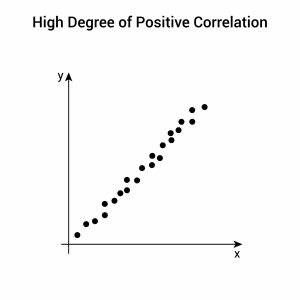• A negative correlation is a relationship between two variables in which an increase in one variable is associated with a decrease in the other. An example of a negative correlation would be the height above sea level and temperature. As you climb the mountain (increase in height), it gets colder (decrease in temperature).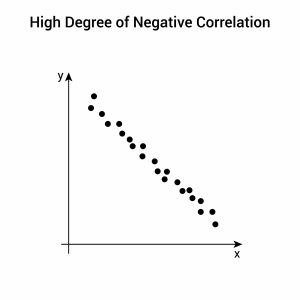• A zero correlation exists when there is no relationship between two variables. For example, there is no relationship between the amount of tea drunk and the level of intelligence.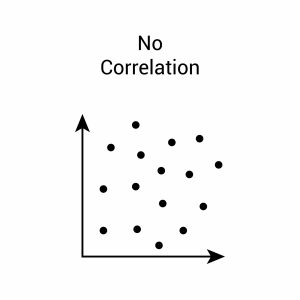## Scatter Plots

A correlation can be expressed visually. This is done by drawing a scatter plot (also known as a scattergram, scatter graph, scatter chart, or scatter diagram).

A scatter plot is a graphical display that shows the relationships or associations between two numerical variables (or co-variables), which are represented as points (or dots) for each pair of scores.

A scatter plot indicates the strength and direction of the correlation between the co-variables.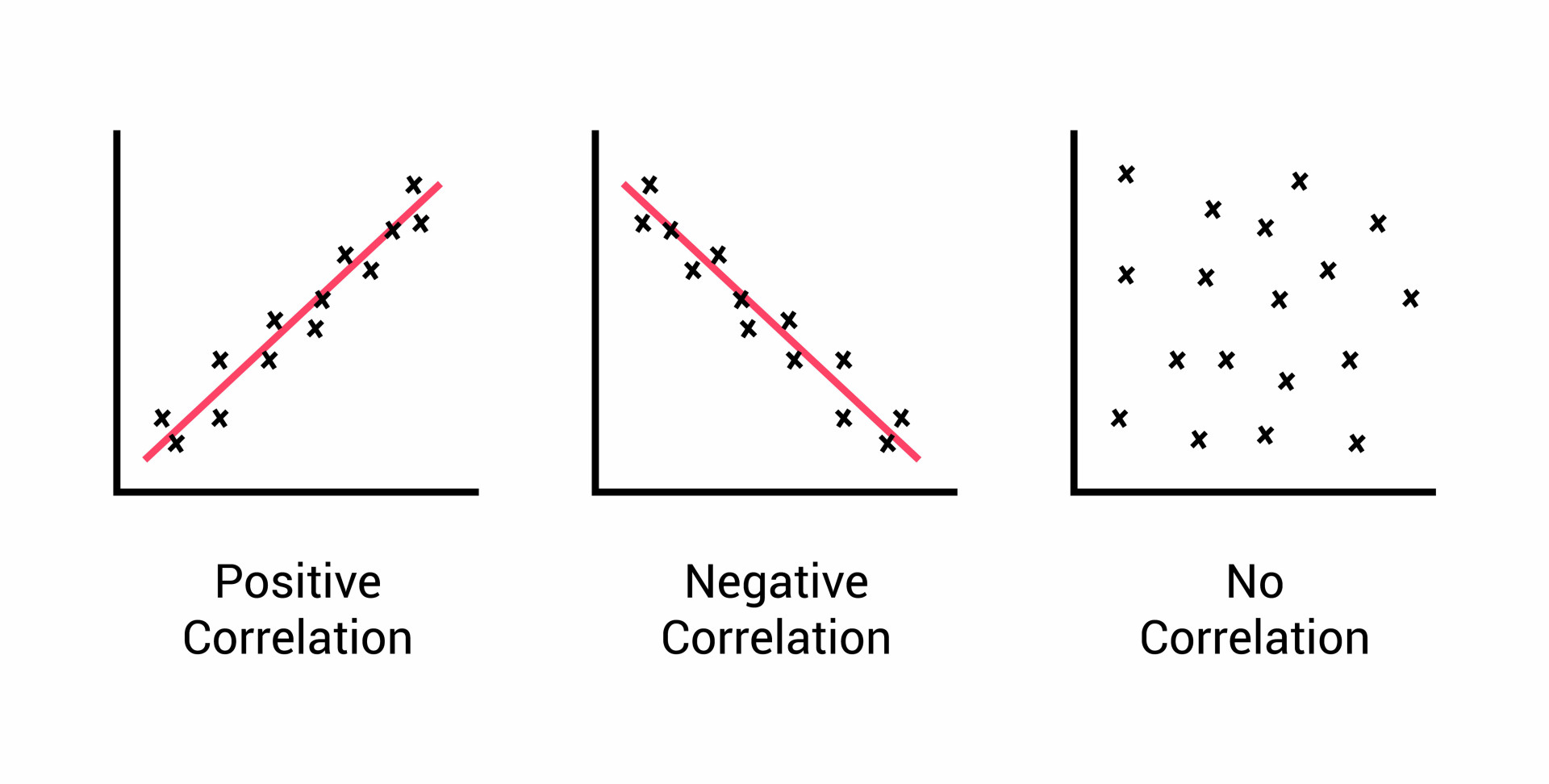When you draw a scatter plot, it doesn’t matter which variable goes on the x-axis and which goes on the y-axis.

Remember, in correlations, we always deal with paired scores, so the values of the two variables taken together will be used to make the diagram.

Decide which variable goes on each axis and then simply put a cross at the point where the two values coincide.

## Uses of Correlations

### Prediction

• If there is a relationship between two variables, we can make predictions about one from another.

### Validity

• Concurrent validity (correlation between a new measure and an established measure).

### Reliability

• Test-retest reliability (are measures consistent?).
• Inter-rater reliability (are observers consistent?).

### Theory verification

• Predictive validity.

## Correlation Coefficients

Instead of drawing a scatter plot, a correlation can be expressed numerically as a coefficient, ranging from -1 to +1. When working with continuous variables, the correlation coefficient to use is Pearson’s r.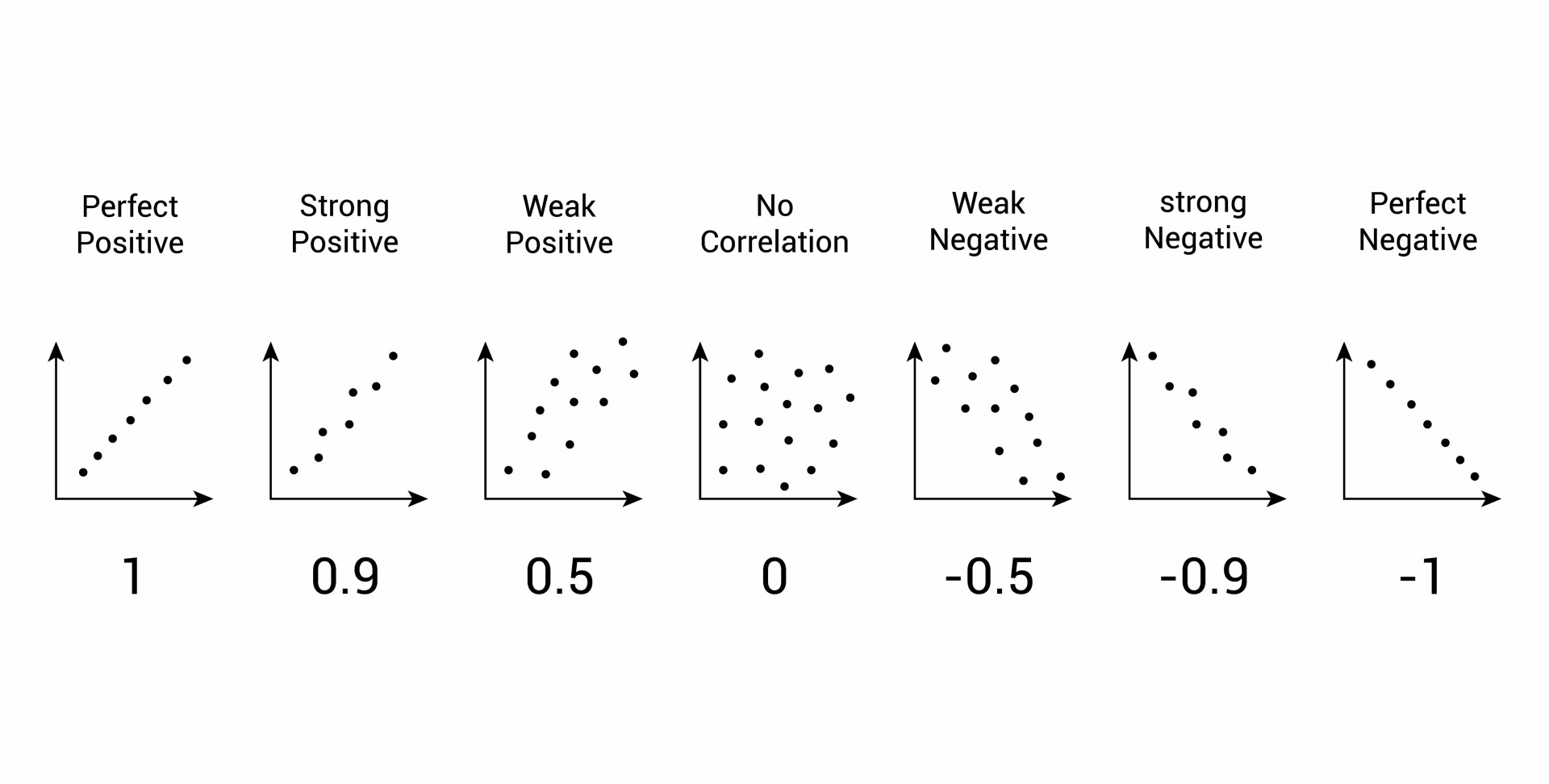The correlation coefficient ( r ) indicates the extent to which the pairs of numbers for these two variables lie on a straight line. Values over zero indicate a positive correlation, while values under zero indicate a negative correlation.

A correlation of –1 indicates a perfect negative correlation, meaning that as one variable goes up, the other goes down. A correlation of +1 indicates a perfect positive correlation, meaning that as one variable goes up, the other goes up.

There is no rule for determining what correlation size is considered strong, moderate, or weak. The interpretation of the coefficient depends on the topic of study.

When studying things that are difficult to measure, we should expect the correlation coefficients to be lower (e.g., above 0.4 to be relatively strong). When we are studying things that are easier to measure, such as socioeconomic status, we expect higher correlations (e.g., above 0.75 to be relatively strong).)

In these kinds of studies, we rarely see correlations above 0.6. For this kind of data, we generally consider correlations above 0.4 to be relatively strong; correlations between 0.2 and 0.4 are moderate, and those below 0.2 are considered weak.

When we are studying things that are more easily countable, we expect higher correlations. For example, with demographic data, we generally consider correlations above 0.75 to be relatively strong; correlations between 0.45 and 0.75 are moderate, and those below 0.45 are considered weak.

## Correlation vs. Causation

Causation means that one variable (often called the predictor variable or independent variable) causes the other (often called the outcome variable or dependent variable).

Experiments can be conducted to establish causation. An experiment isolates and manipulates the independent variable to observe its effect on the dependent variable and controls the environment in order that extraneous variables may be eliminated.

A correlation between variables, however, does not automatically mean that the change in one variable is the cause of the change in the values of the other variable. A correlation only shows if there is a relationship between variables.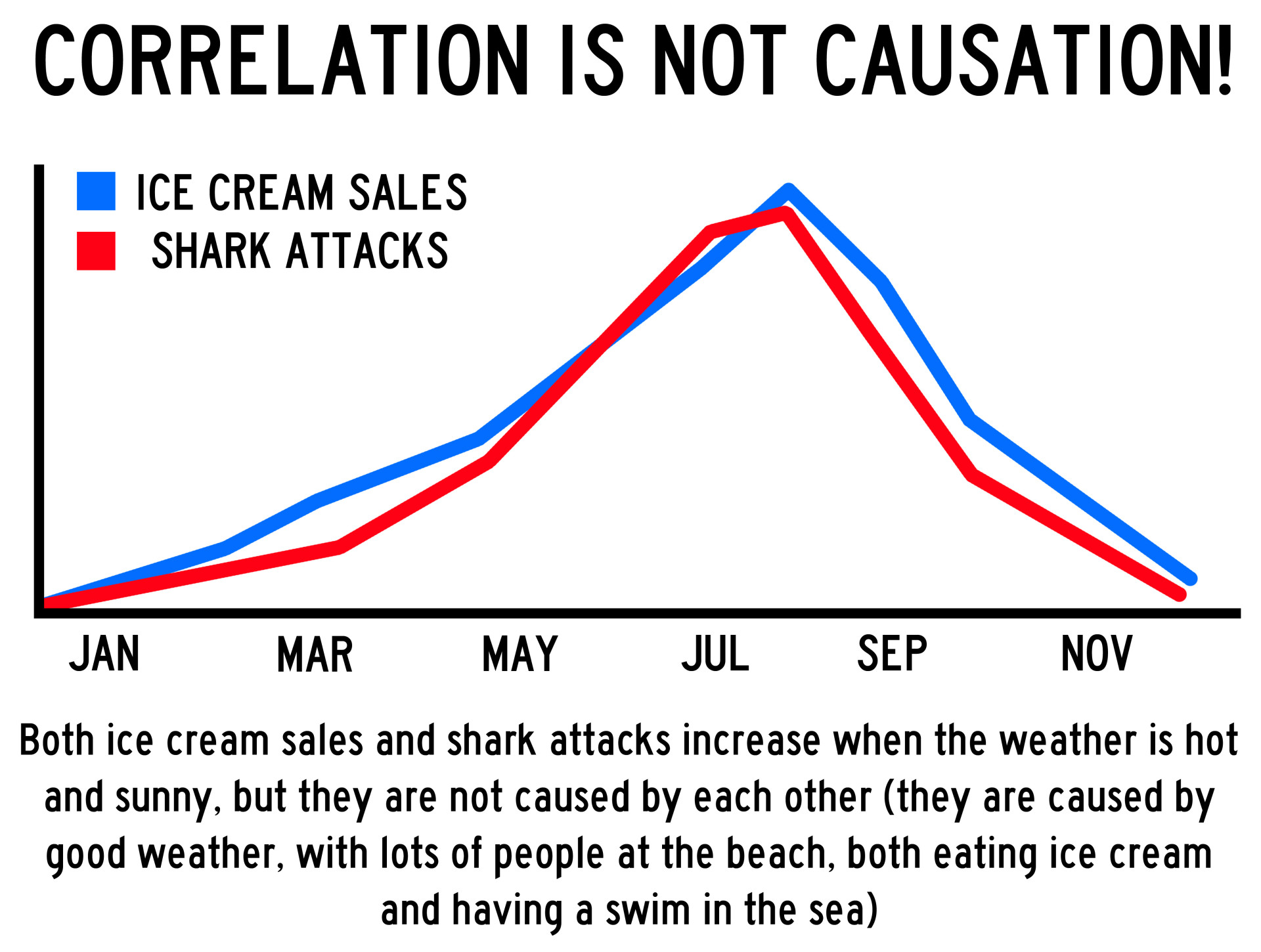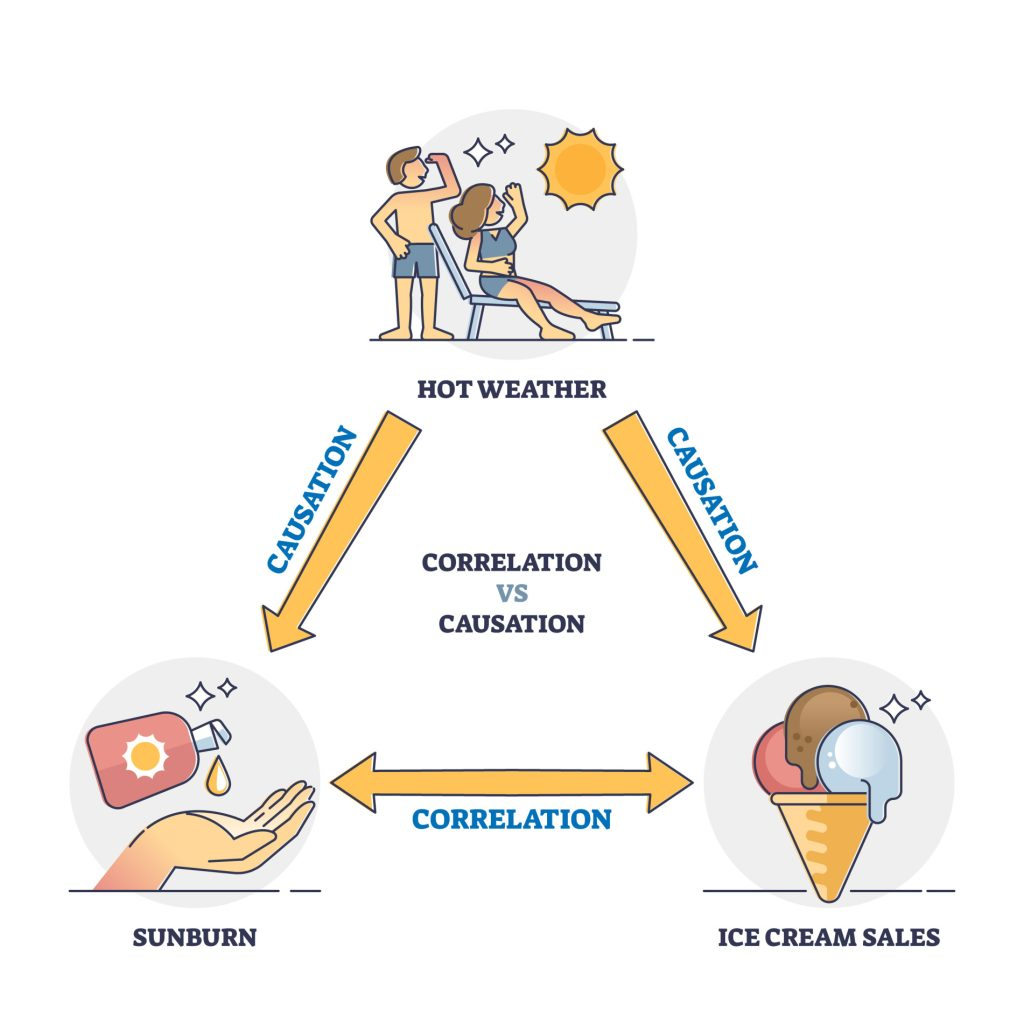While variables are sometimes correlated because one does cause the other, it could also be that some other factor, a confounding variable, is actually causing the systematic movement in our variables of interest.

Correlation does not always prove causation, as a third variable may be involved. For example, being a patient in a hospital is correlated with dying, but this does not mean that one event causes the other, as another third variable might be involved (such as diet and level of exercise).

## Summary

“Correlation is not causation” means that just because two variables are related it does not necessarily mean that one causes the other.

A correlation identifies variables and looks for a relationship between them. An experiment tests the effect that an independent variable has upon a dependent variable but a correlation looks for a relationship between two variables.

This means that the experiment can predict cause and effect (causation) but a correlation can only predict a relationship, as another extraneous variable may be involved that it not known about.

## Strengths

1. Correlation allows the researcher to investigate naturally occurring variables that may be unethical or impractical to test experimentally. For example, it would be unethical to conduct an experiment on whether smoking causes lung cancer.

2 . Correlation allows the researcher to clearly and easily see if there is a relationship between variables. This can then be displayed in a graphical form.

## Limitations

1. Correlation is not and cannot be taken to imply causation. Even if there is a very strong association between two variables, we cannot assume that one causes the other.

For example, suppose we found a positive correlation between watching violence on T.V. and violent behavior in adolescence. It could be that the cause of both these is a third (extraneous) variable – for example, growing up in a violent home – and that both the watching of T.V. and the violent behavior is the outcome of this.

2 . Correlation does not allow us to go beyond the given data. For example, suppose it was found that there was an association between time spent on homework (1/2 hour to 3 hours) and the number of G.C.S.E. passes (1 to 6). It would not be legitimate to infer from this that spending 6 hours on homework would likely generate 12 G.C.S.E. passes.Olivia Guy-Evans

BSc (Hons), Psychology, MSc, Psychology of Education

Associate Editor for Simply Psychology

Olivia Guy-Evans is a writer and associate editor for Simply Psychology. She has previously worked in healthcare and educational sectors.Saul Mcleod, PhD

Educator, Researcher

BSc (Hons) Psychology, MRes, PhD, University of Manchester

Saul Mcleod, Ph.D., is a qualified psychology teacher with over 18 years experience of working in further and higher education.